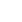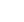• English
• Hindi
• Bengali
• Marathi
• Telugu
• Tamil
• Gujarati
• Odia
• Malayalam

## Tractor Loan EMI Calculator

Calculating your Tractor Loan EMI has never been easier. Use our Tractor Loan EMI Calculator to input your desired amount, interest rate and tenure, and view an instant summary of your EMI amounts. You can also simply adjust the amount and tenure to see how it affects your EMI repayments. Make smart investments for your farm with our Tractor Loan EMI Calculator

1L 1Cr
6% 26%
12 60
Total Amount Payable

• Principal Amount

• Total Amount

Monthly EMI

### Tractor Loan EMI: What Is It?

A Tractor Loan EMI (Equated Monthly Instalment) is the monthly payment you make until your tractor loan is fully repaid. This amount comprises both the principal loan amount and the interest accrued.

### Tractor Loan EMI: How Is It Calculated?

To calculate your Tractor Loan EMI manually, you can use this formula:

EMI = [P x R x (1+R)^N]/[(1+R)^N-1]

• P represents the loan amount.
• R is the monthly interest rate (annual interest rate divided by 12).
• N denotes the loan tenure in months.
For instance, if you take a loan of ₹5 lakhs to buy a tractor at an interest rate of 10% per annum for a tenure of 5 years. The EMI for this loan would be calculated as follows:

EMI = [500000 * 0.00833 * (1 + 0.00833)^60] / [(1 + 0.00833)^60 – 1]

EMI = ₹10,833.33

This means that you would need to pay ₹10,833.33 every month for 5 years to repay the loan

### Benefits of Using a Tractor Loan EMI Calculator

• Speed and convenience: It’s a quick and easy tool.
• Accuracy: Ensures precise EMI calculations.
• Financial planning: Helps you budget for your tractor loan.

### How to Use Mahindra Finance Tractor Loan EMI Calculator?

Using a tractor finance EMI calculator is simple. Just provide three key inputs:

• Loan amount
• Interest rate
• Loan tenure

The calculator will then display your monthly EMI. Feel free to experiment with different loan amounts, interest rates, and tenures to find the most suitable option for your budget